## Build Numbered Lists With The Amazing SEQUENCE Function

The SEQUENCE function is a useful function in Google Sheets. It’s a powerful way to generate numbered lists.

Previously, you had to resort to obscure array formulas like

`=ArrayFormula(row(1:5))`

to get lists of numbers. Things got ugly fast if you wanted to customize these lists.

Thankfully today, we have the SEQUENCE function.

As arguments, you specify: 1) the number of rows, 2) the number of columns, 3) a start value, and 4) a step size.

Arguments 2, 3 and 4 are optional. However, if you want to set them you need to include the previous ones (e.g. if you want to set a step size in argument 4, then you need to set 1, 2 and 3 as well).

Keep this order in mind as you look through the examples below and you’ll soon understand how the function works.

## 1. Ascending list of numbers

`=SEQUENCE(5)`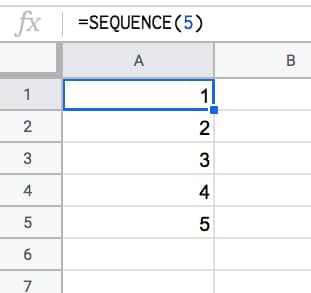## 2. Horizontal list of numbers

Set the row count to 1 and the column count to however many numbers you want e.g. 5:

`=SEQUENCE(1,5)`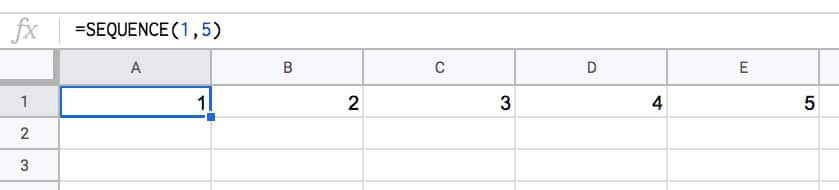## 3. Two-dimensional array of numbers

Set both row and number values:

`=SEQUENCE(10,5)`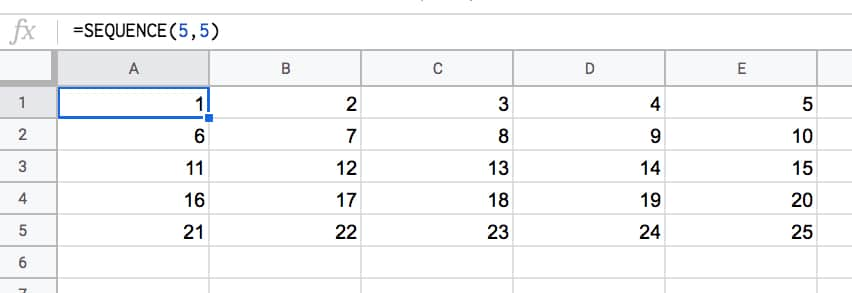## 4. Start from a specific value

Set the third argument to the value you want to start from e.g. 100:

`=SEQUENCE(5,1,100)`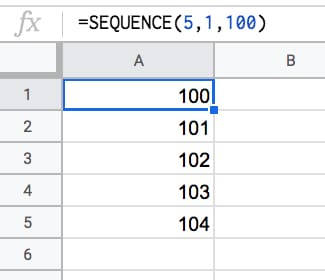## 5. Use a custom step

Set the fourth argument to the size of the step you want to use, e.g. 10:

`=SEQUENCE(5,1,1,10)`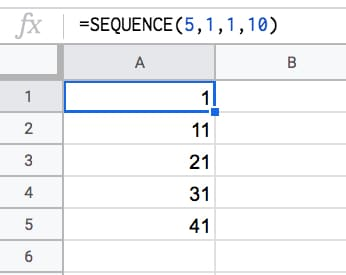## 6. Descending numbers

Set the fourth argument to -1 to count down:

`=SEQUENCE(5,1,5,-1)`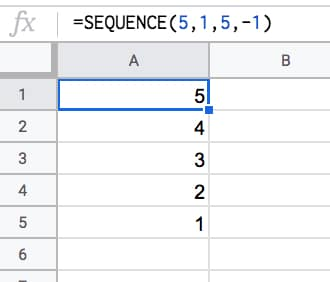## 7. Negative numbers

Set the start value to a negative number and/or count down with negative step:

`=SEQUENCE(5,1,-1,-1)`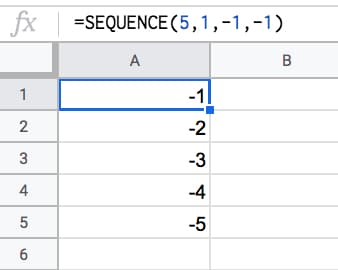## 8. Dates

Dates are stored as numbers in spreadsheets, so you can use them inside the SEQUENCE function. You need to format the column as dates:

`=SEQUENCE(5,1,TODAY(),1)`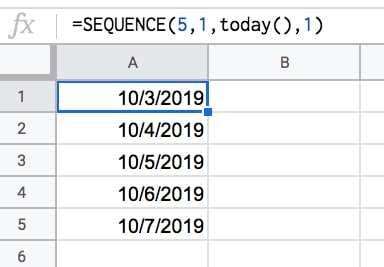## 9. Decimal numbers

Unfortunately you can’t set decimal counts directly inside the SEQUENCE function, so you have to combine with an Array Formula e.g.

`=ArrayFormula( SEQUENCE(5,1,10,1) / 10 )`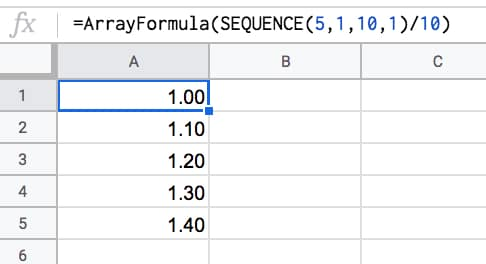## 10. Constant numbers

You’re free to set the step value to 0 if you want an array of constant numbers: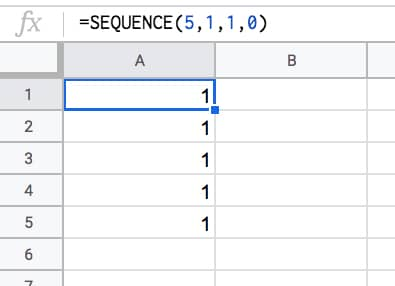## 11. Monthly sequences

Start with this formula in cell A1, which gives the numbers 1 to 12 in a column:

`=SEQUENCE(12)`

In the adjacent column, use this DATE function to create the first day of each month (formula needs to be copied down all 12 rows):

`=DATE(2021,A1,1)`

This can be turned into an Array Formula in the adjacent column, so that a single formula, in cell C1, outputs all 12 dates:

`=ArrayFormula(DATE(2021,A1:A12,1))`

Finally, the original SEQUENCE formula can be nested in place of the range reference, using this formula in cell D1:

`=ArrayFormula(DATE(2021,SEQUENCE(12),1))`

This single formula gives the output:

1/1/2021
2/1/2021
.
.
.
12/1/2021

It’s an elegant way to create a monthly list. It’s not dependent on any other input cells either (columns A, B, C are working columns in this example).

With this formula, you can easily change all the dates, e.g. to 2022.

Building in steps like this a great example of the Onion Method, which I advocate for complex formulas.

## 12. Text and Emoji sequences

You can use a clever trick to set the SEQUENCE output to a blank string using the TEXT function. Then you can append on a text value or an emoji or whatever string you want to create a text list.

For example, this repeats the name “Ben Collins” one hundred times in a column:

`=ArrayFormula(TEXT(SEQUENCE(100,1,1,1),"")&"Ben Collins")`

And, by using the CHAR function, you can also make emoji lists. For example, here’s a 10 by 10 grid of tacos:

`=ArrayFormula(TEXT(SEQUENCE(10,10,1,1),"")&CHAR(127790))`Have you got any examples of using the SEQUENCE function?

## Unpivot In Google Sheets With Formulas (How To Turn Wide Data Into Tall Data)

Unpivot in Google Sheets is a method to turn “wide” tables into “tall” tables, which are more convenient for analysis.

Suppose we have a wide table like this: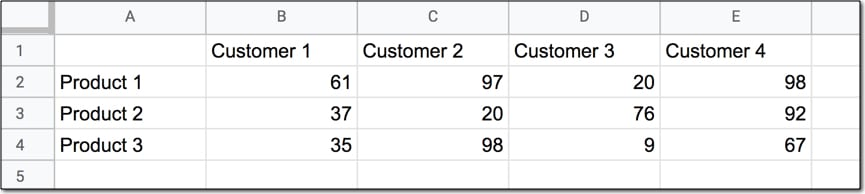Wide data like this is good for the Google Sheets chart tool but it’s not ideal for creating pivot tables or doing analysis. The main reason is that data is captured in the column headings, which prevents you using it in pivot tables for analyis.

So we want to transform this data — unpivot it — into the tall format that is the way databases store data: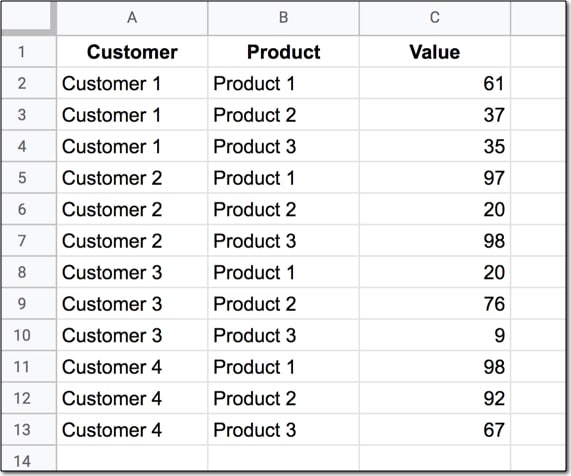But how do we unpivot our data like that?

It turns out it’s quite hard.

It’s harder than going the other direction, turning tall data into wide data tables, which we can do with a pivot table.

This article looks at how to do it using formulas so if you’re ready for some complex formulas, let’s dive in…

We’ll use the wide dataset shown in the first image at the top of this post.

The output of our formulas should look like the second image in this post.

In other words, we need to create 16 rows to account for the different pairings of Customer and Product, e.g. Customer 1 + Product 1, Customer 1 + Product 2, etc. all the way up to Customer 4 + Product 4.

Of course, we’ll employ the Onion Method to understand these formulas.

### Template

Feel free to make your own copy (File > Make a copy…).

(If you can’t open the file, it’s likely because your G Suite account prohibits opening files from external sources. Talk to your G Suite administrator or try opening the file in an incognito browser.)

### Step 1: Combine The Data

Use an array formula like this to combine the column headings (Customer 1, Customer 2, etc.) with the row headings (Product 1, Product 2, Product 3, etc.) and the data.

It’s crucial to add a special character between these sections of the dataset though, so we can split them up later on. I’ve used the fox emoji (because, why not?) but you can use whatever you like, provided it’s unique and doesn’t occur anywhere in the dataset.

`=ArrayFormula(B1:E1&"🦊"&A2:A4&"🦊"&B2:E4)`

The output of this formula is: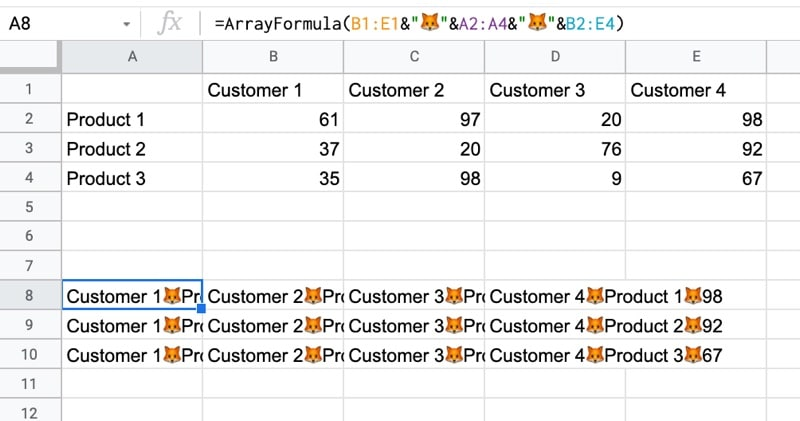### Step 2: Flatten The Data

Before the introduction of the FLATTEN function, this step was much, much harder, involving lots of weird formulas.

Thankfully the FLATTEN function does away with all of that and simply stacks all of the columns in the range on top of each other. So in this example, our combined data turns into a single column.

`=ArrayFormula(FLATTEN(B1:E1&"🦊"&A2:A4&"🦊"&B2:E4))`

The result is: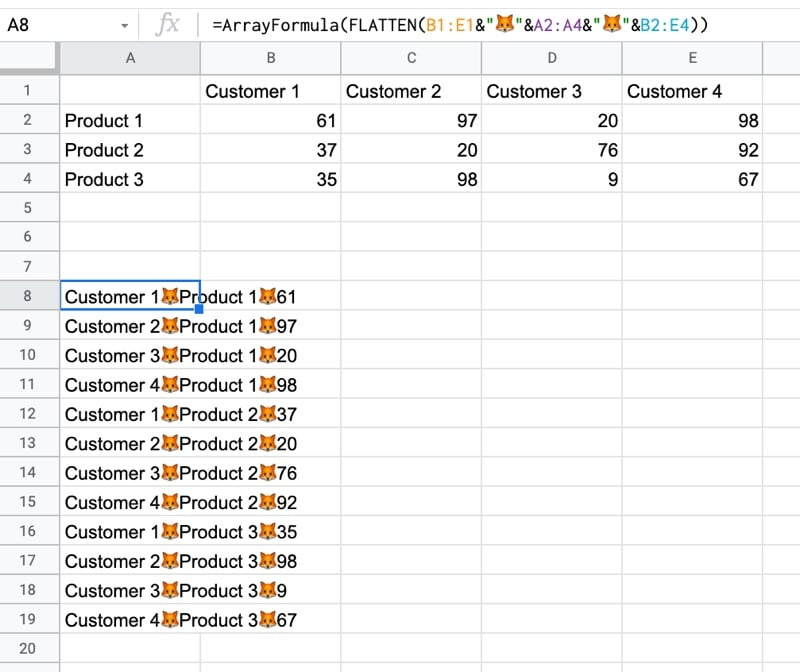### Step 3: Split The Data Into Columns

The final step is to split this new tall column into separate columns for each data type. You can see now why we needed to include the fox emoji so that we have a unique character to split the data on.

Wrap the formula from step 2 with the SPLIT function and set the delimiter to “🦊”:

`=ArrayFormula(SPLIT(FLATTEN(B1:E1&"🦊"&A2:A4&"🦊"&B2:E4),"🦊"))`

This splits the data into the tall data format we want. All that’s left is to add the correct column headings.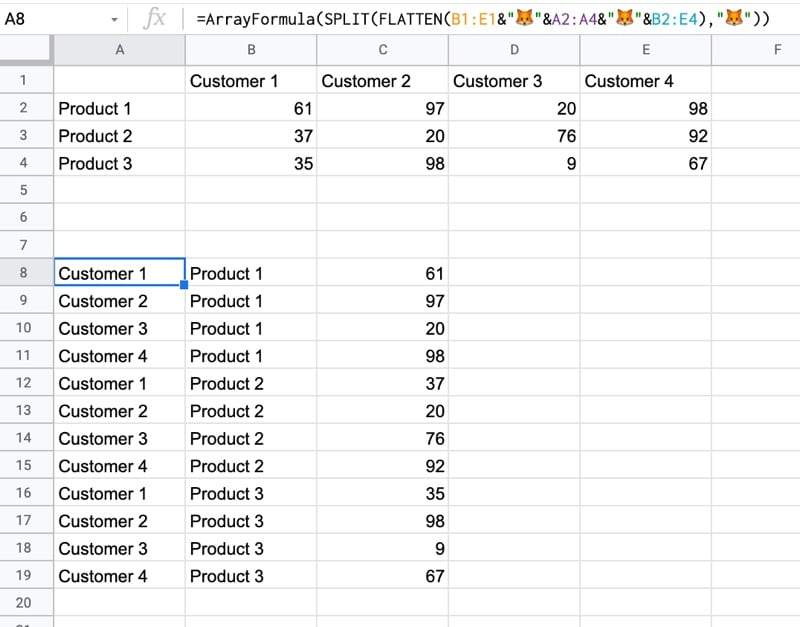## Unpivot With Apps Script

You can also use Apps Script to unpivot data.

Have a look at the example sheet from the first answer of this Stack Overflow post.

## How Do Array Formulas Work In Google Sheets?

Array Formulas have a fearsome reputation in the spreadsheet world (if you’ve even heard of them that is).

Array Formulas allow you to output a range of cells, rather than a single value. They also let you use non-array functions with arrays (think ranges) of data.

In this post I’m going to run through the basics of using array formulas, and you’ll see they’re really not that scary.

Hip, Hip Array!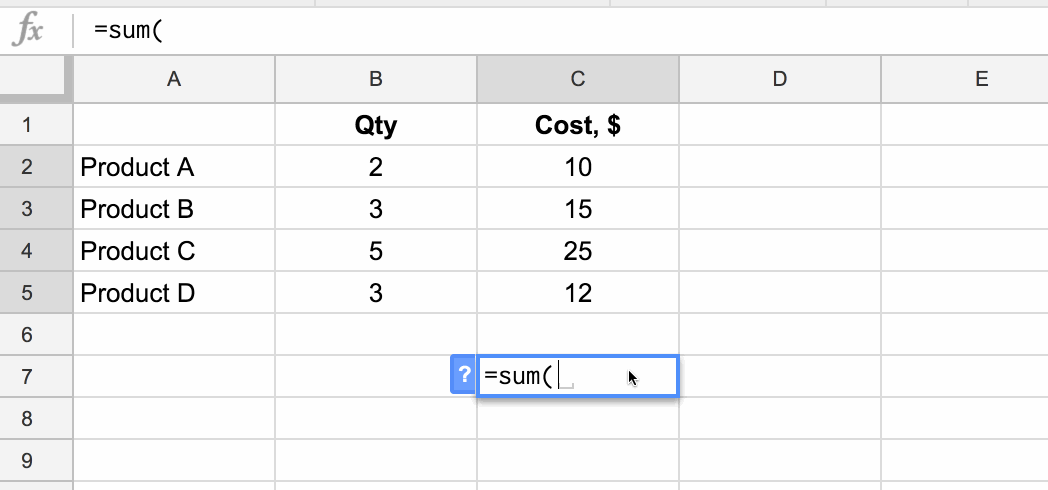## What are array formulas in Google Sheets?

First of all, what are they?

To the uninitiated, they’re mysterious. Elusive. Difficult to understand. Yes, yes, and yes, but they are incredibly useful in the right situations.

Per the official definition, array formulas enable the display of values returned into multiple rows and/or columns and the use of non-array functions with arrays.

In a nutshell: whereas a normal formula outputs a single value, array formulas output a range of cells!

The easiest way to understand this is through an example.

Imagine we have this dataset, showing the quantity and item cost for four products: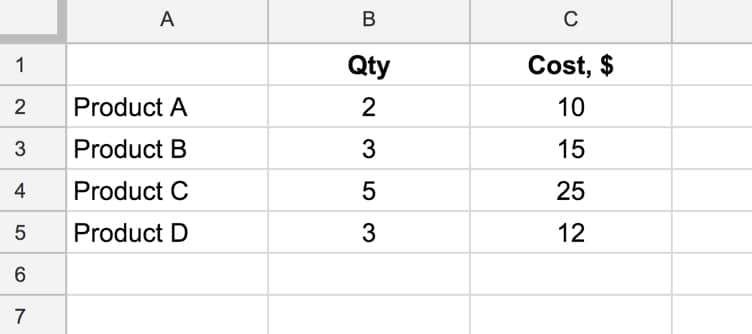and we want to calculate the total cost of all four products.

We could easily do this by adding a formula in column D that multiplies B and C, and then add a sum at the bottom of column D.

However, array formulas let us skip that step and get straight to the answer with a single formula.

### What’s the formula?

`=ArrayFormula(SUM(B2:B5 * C2:C5))`

### How does this formula work?

Ordinarily, when we use the multiplication (*) operator in a Sheet, we give it two numbers or two cells to multiply together.

However, in this case we’re giving it two ranges, or two arrays, of data:

`= B2:B5 * C2:C5`

However, when we hit Enter this gives us a #VALUE! error as shown here: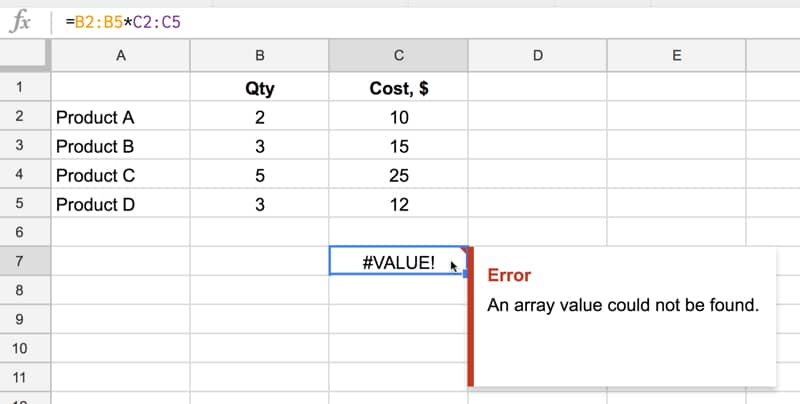We need to tell Google Sheets we want this to be an Array Formula. We do this in two ways.

Either type in the word `ArrayFormula` and add an opening/closing brackets to wrap your formula, or, more easily, just hit Ctrl + Shift + Enter (Cmd + Shift + Enter on a Mac) and Google Sheets will add the ArrayFormula wrapper for us.

`=ArrayFormula(B2:B5 * C2:C5)`

Now it works, and Google Sheets will output an array with each cell corresponding to a row in the original arrays, as shown in the following image:

Effectively what’s happening is that for each row, Google does the calculation and includes that result in our output array (here showing the equivalent formulas):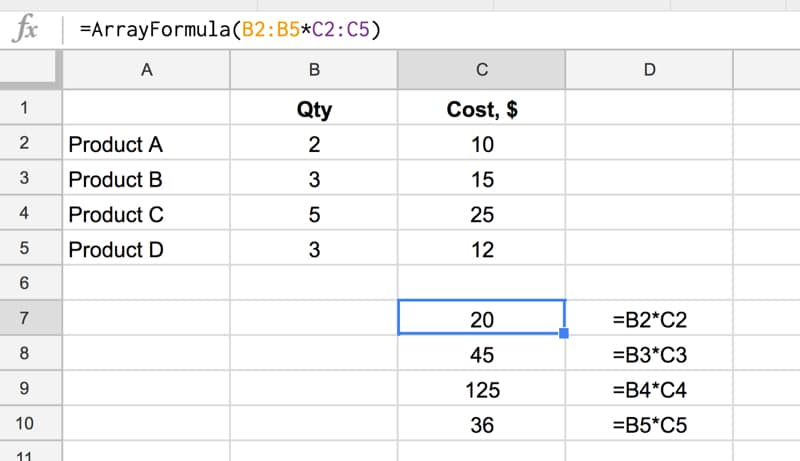and another view, showing how the calculation is performed (just for the first and last row):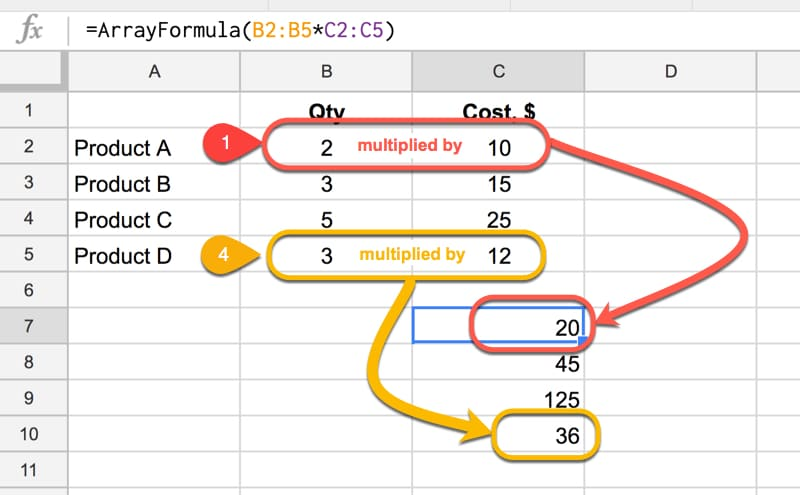Note: array formulas only work if the size of the two arrays match, in this case each one has 4 numbers, so each row multiplication can happen.

Finally, we simply include the SUM function to add the four numbers:

`=ArrayFormula(SUM(B2:B5 * C2:C5))`

as follows:Quick Aside:

This calculation could also be done with the SUMPRODUCT formula, which takes array values as inputs, multiplies them and adds them together:

`=SUMPRODUCT(B2:B5 , C2:C5)`

### Another Array Formula Multiplication Example

In this example, we enter a single formula to multiply an array of row headings against an array of column headings.

This only works because the dimensions of the arrays are compatible, since the number of columns in the first matrix is equal to the number of rows in the second matrix: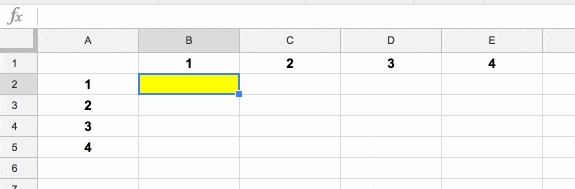### Array Formula With IF Function

This is an example of a non-array function being used with arrays (ranges). It works because we designate the IF formula as an Array Formula.

Consider a standard IF statement which checks whether a value in column A is over \$2,000 or not:

`=IF(A2>2000,"Yes","No")`

This formula would then be copied into each row where we want to run the test.

We can change this to a single Array Formula at the top of the column and run the IF statement across all the rows at once. For example, suppose we had values in rows 2 to 10 then we create a single Array Formula like this:

`=ArrayFormula(IF(A2:A10>2000,"Yes","No"))`

This single formula, on row 2, will create an output array that fills rows 2 to 10 with “Yes”/”No” answers, as shown in the following image: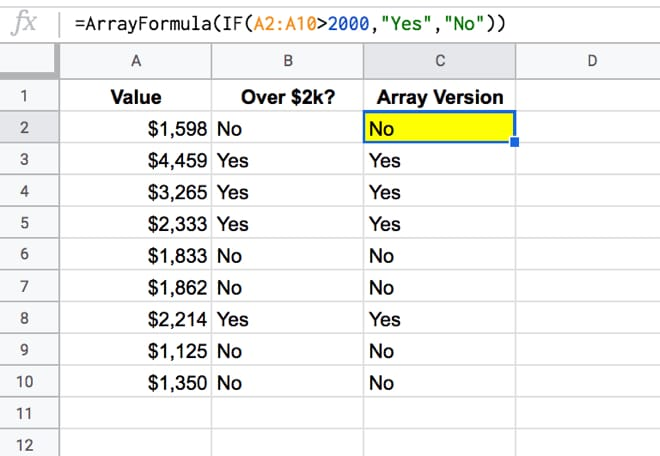## How to add a total row to a Query Function table in Google Sheets

This article looks at how to add a total row to tables generated using the Query function in Google Sheets. It’s an interesting use case for array formulas, using the `{...}` notation, rather than the `ArrayFormula` notation.

So what the heck does this all mean?

It means we’re going to see how to add a total row like this:

using an array formula of this form:

`= { QUERY ; { "TOTAL" , SUM(range) } }`

Now of course, at this stage you should be asking:

“But Ben, why not just write the word `TOTAL` under the first column, and `=SUM(range)` in the second column and be done with it?”

Well, it’s a good question so let’s answer it!

The reason for using this method is because the total line is added dynamically, so it will be appended directly at the end of the table, and won’t break if the table expands or contracts, if more data is added.

It’ll always move up or down, so it sits there as the final row.